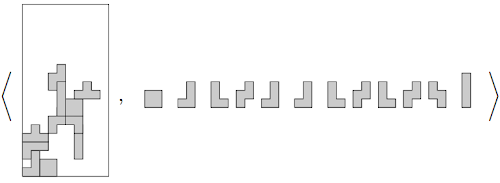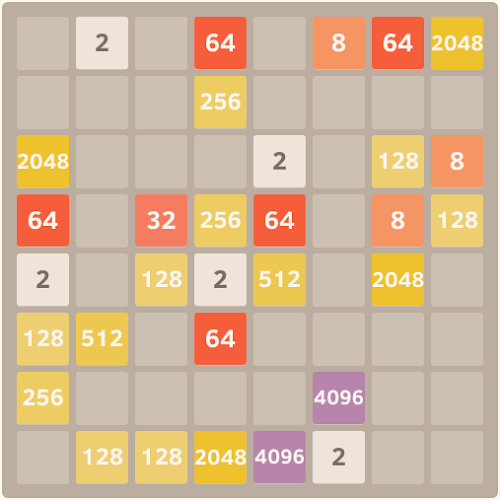## Thursday, March 27, 2014

### 2048 is in NP

(This post assumes some familiarity with the game.)

### Turning interactive games into pen/paper puzzles

In computer science, $NP$ is the collection of all the types of pen/paper puzzles whose solutions are easy to double-check (but not necessarily easy to find). These span a wide range of difficulties, from mazes to Sudoku to who owns the zebra?-style logic puzzles.

(Formally, $NP$ is the set of decision problems for which yes answers can be verified in polynomial time.)

Of course, 2048 is a Category 1 life-threatening memetic contagion video game, not a pen/paper puzzle. So we're going to need to do a little bit of massaging to talk about it as if it was.

That's where Tetris comes in.Chen, C. Rectangular grids and puzzle game addiction: a journey into the collective human subconscious. Dissertation. Published Jun 2048.

In the game of Tetris, players rotate and drop tetrominoes (four-block pieces) into a vertical grid. They progress by filling (and thus clearing) entire rows of blocks; they lose when the grid is too cluttered to place anything else. To add to the challenge, they are unable to predict what tetrominoes they will be given more than a turn or two in the future: thus they must plan defensively against this element of randomness.

First submitted to arXiv in 2002, Demaine, Hohenberger and Liben-Nowell's paper Tetris is Hard, Even to Approximate [DHL03] analyses Tetris in the language of computational complexity. To massage Tetris into the form of a pen/paper puzzle decision problem, they make the following changes to the standard game rules:

• Initial state — The board begins pre-filled.
• No unpredictability — The player is told, in advance, the entire sequence of pieces that will rain from the sky.

This makes it possible to succinctly describe interesting instances of the game.An example instance of pen/paper Tetris. On the left, a starting game board, with a particular size and pieces already placed. On the right, the sequence of tetrominoes the player will receive. The angle brackets and tuple notation are for mathematical street cred. Taken from these lecture slides by Susan Hohenberger.

Of course, for this to be a true pen/paper puzzle decision problem, we need an actual yes/no question to solve. The authors propose several, including:

• Can you clear $x$ rows?
• Can you survive $x$ turns?
• Can you achieve $x$ 4-row combos?

But enough of Tetris. Let's try applying this approach to 2048.

In the game of 2048, players move tiles around a grid, on each turn picking a direction and pushing all tiles as far as they will go. They progress by merging identical tiles together to create higher-value tiles; they lose when they are no longer able to move. To add to the challenge, new low-value tiles are added to the board at random after each turn: thus they must plan defensively against this unpredictability.

As per Tetris, we make a few changes to the rules of 2048 to turn this into a legitimate pen/paper puzzle:

• Initial state — The board begins pre-filled.
• No unpredictability — No new pieces will be added to the board.

Thus a sample game instance might look like this:An example instance of pen/paper 2048. A board, with a particular size and tiles already placed. No extra tiles will be added. Styling taken from CyberZHG's mod, http://cyberzhg.github.io/2048/.

As for the corresponding yes/no question, I propose the following:

• Can you merge all the pieces into a single tile?
• Can you merge all the pieces into distinct types of tile?
• Can you make at least $x$ merges?
• Can you score at least $x$ points?

Of course, nothing is stopping us from asking weirder questions, such as Is there a sequence of moves that, in Morse code, spells out a solution to the following Sudoku grid...?. But the ones I have listed above are all fairly natural questions that arise from the nature of the 2048 game itself. In fact, they have an additional property which I'm going to dub merge-based.

[Highly informal] definition: A merge-based 2048 pen/paper puzzle decision problem is one which starts with a game instance of 2048 and asks a question...

• ...which can be answered in the affirmative by a sequence of moves,
• ...and where the only thing about this sequence of moves that matters is what types of merge happened (e.g. one 2+2=4 merge and three 128+128=256 merges).

Now we have everything we need to formalise the title of this post!

Main Theorem: All merge-based 2048 decision problems are in $NP$. That is, affirmative answers to these puzzles can be double-checked quickly.

### That seems trivial...

...all you have to do is write out a sequence of moves that solves the puzzle. It's just like how you'd write out the move sequence for Tetris, or fill out the grid for Sudoku.

Well, yes, hypothetical algorithms-professor interlocutor, but the fact that you can get away with this isn't actually as trivial as it looks at first glance.

The tricky part here is that we don't actually know how long the move sequence is going to be. Part of the definition of $NP$ is that the double-checking process has to be fast (polynomial) relative to the size of the input, not the size of the certificate filled-in solution. So a gargantuan (e.g. exponential) sequence of moves, even one that only has to be read through once, won't cut it.

An example where this comes to bite us is the famous Towers of Hanoi puzzle. Consider the question: Can you move all the pieces from the first peg to the second peg? As it happens, the answer is always yes, regardless of starting tower size, but the number of moves required is $2^n-1$: far too many to squeeze into the answers section at the back of a newspaper, and far too fast-growing for this to be a useable $NP$ certificate.

(In games like Chess or Go, the proof that black has a winning position may be exponentially large relative to the board size. Not coincidentally, Sudoku puzzles tend to appear in newspapers more often than white to mate in 90 puzzles.)

In the Tetris variant from before, Demaine et al. showed that move sequences were polynomial in size, because each piece can only move with the width and height of the gameboard before coming to a standstill. (Thus the number of moves is bounded by the size of the gameboard, times the number of pieces: this is polynomial in the input size.) We haven't yet proven any such guarantees for 2048.

Thus we really need to prove:

Lemma 1 (small number of moves): All yes answers to merge-based 2048 decision problems involve a small (i.e. polynomial) number of moves.

Proof (sketch): Observe that:

• A polynomial number of merges happen.
• There only needs to be a polynomial number of moves between any two merges.

Of course, this is simply begging the question: are these observations easy to prove?

As it happens, the first observation is easy: The number of merges that happen in a sequence of moves is less than the initial number of pieces on the board. This is because every time we merge two tiles, the total number of tiles on the board decreases. If we start with $n$ tiles, we get to make at most $n-1$ merges.

As for the second observation... well, here's where things get fun. Since our decision problems are merge-based, we know that we don't get anything out of messing around (e.g. pressing the up key a billion times) between merges. We need to prove something like the following:

Lemma 2 (small number of moves between merges): Given a starting game instance, and an arbitrarily long sequence of moves-without-merges ending in a merge, there is a short (i.e. polynomial) sequence of moves that (merges the same tiles and) gives the same ending state.

Our strategy to prove this is going to work by collapsing the ridiculously long sequence of moves into a much smaller form. To start off with, notice the following:

• L, L has the same effect as L. (By our assumption, the first L-move doesn't create a merge, so the second doesn't do anything.)
• U, D has the same effect as D.

This allows us to collapse out long runs of up/down, left/right, and other presses, until we're left with a sequence of moves which alternates between [either up or down] and [either left or right] moves. Excellent. But this still may not be polynomially-bounded.

Next step:

• D, L, D has the same effect as D, L. (The first two moves push the tiles into the bottom-left corner. Hence the last move doesn't do anything.)

This observation allows us to get rid of even more moves from our arbitrarily long sequence. The only possible follow-up move to D, L is U. Thus we know that our sequence of moves looks something like this:

• ..., D, L, U, R, D, L, U, R, ... (i.e. clockwise rotation)
• ..., R, U, L, D, R, U, L, D, ... (i.e. anticlockwise rotation)

This is fascinating in of itself, because it means that, once we factor in the player's choice of first move, there are only (up to) eight distinct strategies one can take between merges. An efficient overall game strategy thus consists of picking clockwise or anticlockwise, mashing the buttons in that order until two tiles merge, and repeating as desired.

Can we now show that we only need to rotate around clockwise for a polynomial amount of time?

The above diagram shows how the position of tiles on a board changes after a non-merging move. But there is a more useful way to view the board!

Imagine that every time we press up or down, our view of the board flips horizontally, and every time we press left or right, our view of the board flips vertically. Then when the tiles all start out packed in the top-left (or any other corner, as they will after the first two moves), the overall shape formed by the tiles doesn't change.The effect of the same D move, after flipping the board. Notice how the effect has been to individually flip the contents of each column.

From this projection of the board, we can see that the effect of a vertical move is to flip the individual columns vertically. Similarly, the effect of a horizontal move is to flip the individual rows horizontally.

Thus tiles move around on predictable orbits like so:

More to the point, the two particular tiles which will end up merging move along on these orbits. Since the orbits have length $\leq n^2$ ($n$ being the board width), the LCM of their orbit lengths is $\leq n^4$. So since they eventually end up adjacent to one another (then merging), this must happen in the first $n^4$ moves.

This means that the amount of time between any two merges is polynomially bounded!

This proves Lemma 2, which completes the proof of Lemma 1, which implies the main theorem.

(I'm not going to insert the you win! screen from Doge2048 here, but feel free to imagine it right now. Savour that smell of many victory.)

### Open problems

• Are these problems in $P$? In $coNP$? $NP$-complete?
• Can all reachable configurations be reached in a polynomial number of moves? (Equivalently: if merges aren't possible and we start spiralling around, will we get back to the start within a polynomial number of moves?)
• Is the 3D version of this problem in $NP$? (There are no longer forced moves in quite the same sense, which breaks our notion of orbits.)

References:
[DHL03] Demaine, Erik D., Susan Hohenberger, and David Liben-Nowell. "Tetris is hard, even to approximate." Computing and Combinatorics. Springer Berlin Heidelberg, 2003. 351-363.
P vs NP course notes by Scott Aaronson, retrieved from here on 26/3/2014.